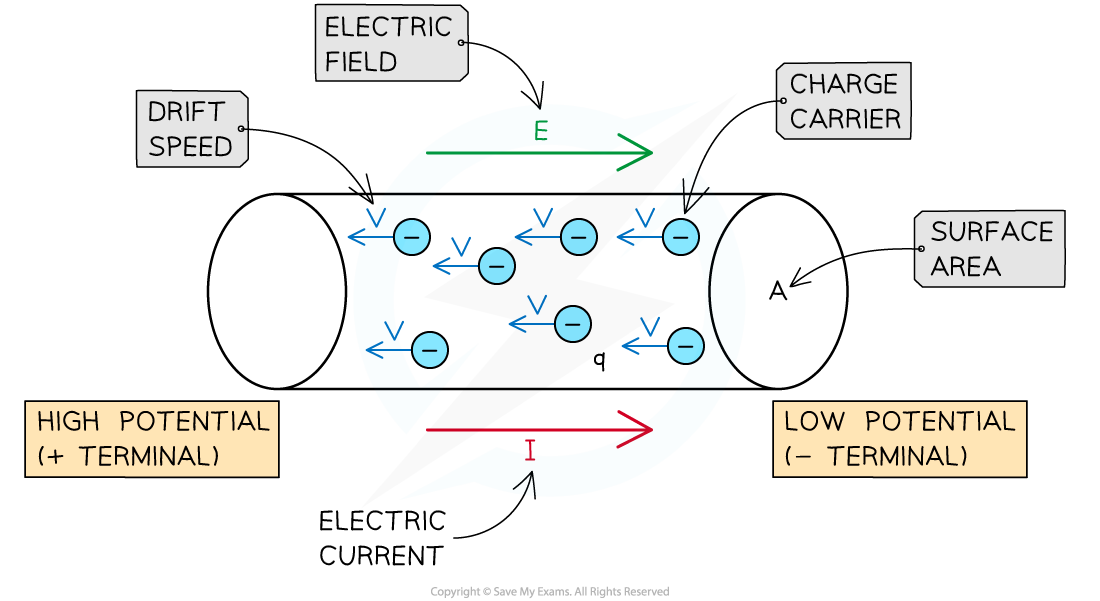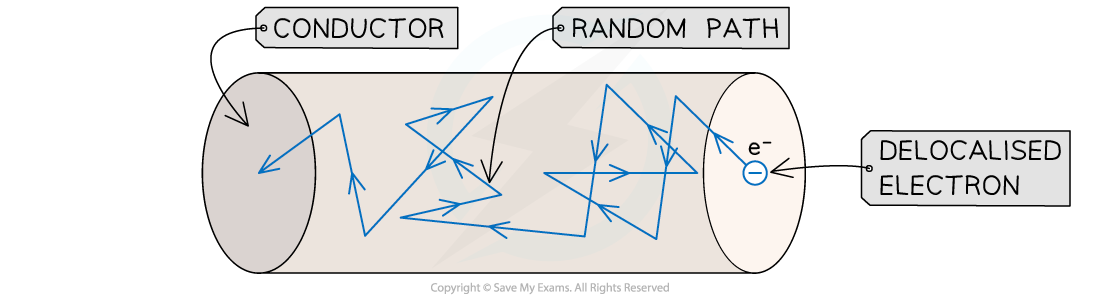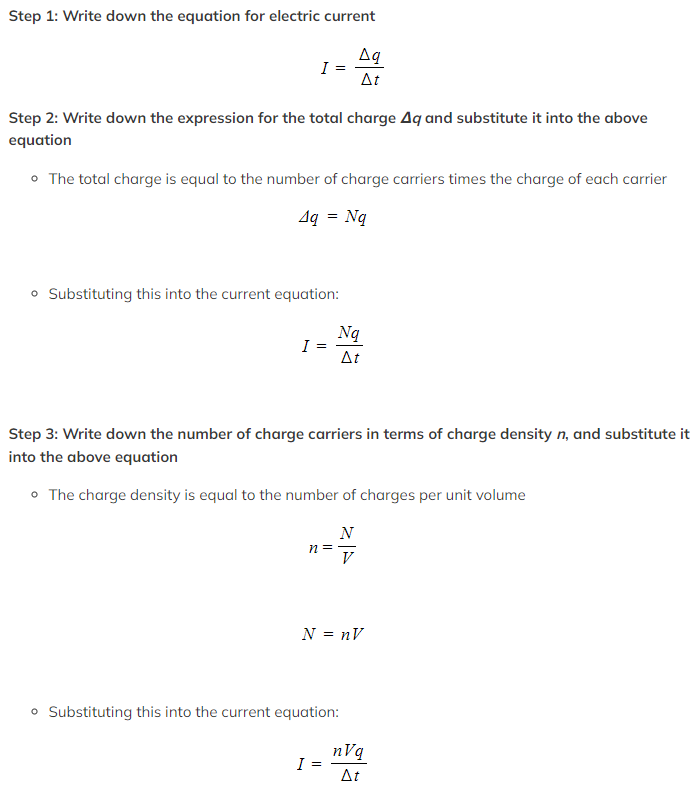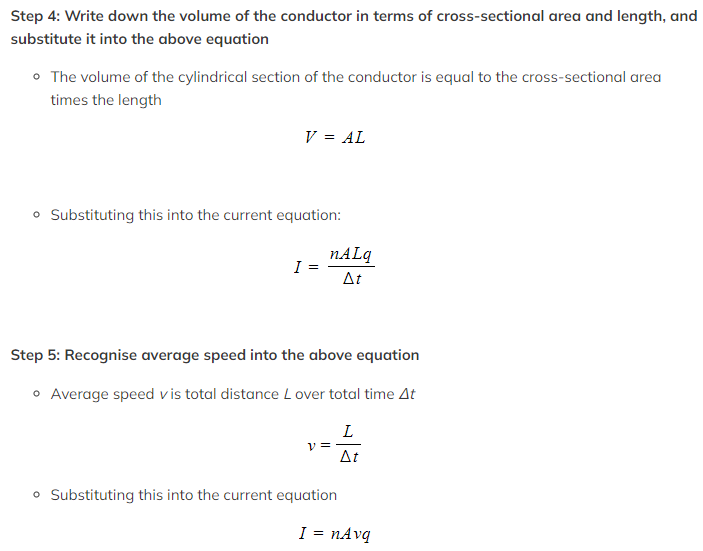# IB DP Physics: SL复习笔记5.1.2 Drift Speed

### Drift Speed

• In conductors, only negatively charged (delocalised) electrons are allowed to move between atoms
• In general, an electric current can arise from the flow of either positive or negative particles
• Charged particles moving through a material or through vacuum are known as charge carriersCharge carriers drift towards the positive terminal of the conductor. Conventional current flows in the opposite direction

#### Movement in a Conductor

• Delocalised electrons are the charge carriers in a conductor
• These electrons normally move randomly through the conductorRandom path of a delocalised electron through a length of conductor

• If a potential difference is applied between two points in the conductor, an electric field is created
• As a consequence:
• An electric force will act on the charge carriers
• The charge carriers will drift along the conductor
• A steady average current will flow through the conductor

#### Electric Current & Drift Speed

• The average speed at which the charge carriers move through a conductor is known as the drift speed
• The value of the drift speed is usually very small
• For most everyday situations, v ∼ 10–4 m s–1
• The electric current arising from the movement of a given number of charge carriers through a conductor is calculated as follows:

I = nAvq

• Where:
• n = number of charge carriers per unit volume, i.e. charge density (m–3)
• A = cross-sectional area of the conductor (m2)
• v = average drift speed of the charge carriers (m s–1)
• q = charge of the charge carriers (C)

#### Worked Example

A number N of charge carriers, each with a charge q, moves through a conductor of length L and cross-sectional area A. Show that the electric current flowing through the conductor is given by:

I = nAvq

Where n is the number of charge carriers per unit volume and v is their drift speed.